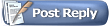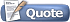Finance & Money ForumsNeed help calculating APR with limited info

 Register FAQ Members List Calendar Search Today's Posts Mark Forums Read

 Loans Discussions about loans and lending practices.ArtoriusJunior Member Join Date: Nov 2007 Posts: 1Need help calculating APR with limited info

Hoping to draw on the help of an APR genius on this challenge that so far Excel / Finance and Mathematicians are struggling to find a solution on.

I have 5,000 loan details that I need to calculate the APR for. However, I only have the following information:-

Original Loan Amount (i.e. £10,000)

Term of Loan (i.e. 120 months)

Total Payable (i.e. £13,000)

Question is how can I work out the APR based solely on this information? I am trying to solve this using Excel, data being columns A to C. In column D, I want the APR to be calculated.

One person suggested calculating the IRR and using that but I do not have the monthly payment amount for these loans, only the starting and end point.

Anyone help with this .... you're my last hope of saving my bacon ..... thank you :-)paulmeadeJunior Member Join Date: Oct 2008 Location: Bournemouth Posts: 8Re: Need help calculating APR with limited info

5.96 AprbrianAdministrator Join Date: Mar 2006 Posts: 788Re: Need help calculating APR with limited info

I was under the impression the company would have to provide clear information on APR - so if you don't have it, worth asking them for it.Markuk1972Finance Market forums Join Date: Dec 2008 Posts: 1Re: Need help calculating APR with limited info

This is a tough one.

APR is based on the total cost of all fees and charges. This differs between each financial institution and the way they calculate the APR will differ from company to company.

Each company is obliged to send you a full calculation to how they reach your apr.loantimeJunior Member Join Date: Jan 2009 Posts: 8Re: Need help calculating APR with limited info

Remember that it's not as simple as taking the amount of time and the original cost, because interest is compound. Let me explain:

With your starting value of 10,000 (I'll ignore currency symbols to make it easier) and with an interest rate of 10% (for example), after 1 year you would have 11,000. But! the year after that you would have 12,100, an actual increase from the original value of 21%, not 20%. So, for every year you add on, you have to sum the power of the original APR. So, in the third year, you'd have 13,310, which is an increase of 33.1% from the original value (APR*3 + 3.1%) now the hard part comes when you have to work out the 3.1% value. Also note that last year, this value was only 1%. So it doesn't seem to be increasing at a steady rate. That's correct, you have to compound it.

But wouldn't it be easier if there was just a formula to do this? Well there is. Essentially, look at the mathematical operation you have to do to get the final answer. You start off with 1,000 and need to increase it by 10%, which is 110% of the original value. Convert this to decimal and you get 1.1 . This will become our magic number for a bit.

Consider 1000 * 1.1 = 1100, then 1100 * 1.1 = 1210, which is actually the same as 1000 * (1.1*1.1) = 1210 . Now you can see how quickly we will come up with the following formula:
t = number of years
x = starting value
a = apr
y = final amount
y = x * (a^t)

Now, that's pretty simple, right? Now the problem is doing it backwards. We're missing a, but we have all the other values. So using the example you gave us:
x = 10000, t = 10, y = 13000
Which will give us:
13000 = 10000 * (a^10)
So, we need to rearrange this to get:
13000/10000 = a^10 or 1.3 = a^10
Now, just rearrange the equation:
a = 10th root of 1.3
So the formula would be:
apr = nth root of (total repayment / starting amount)

Hope that helped!

Last edited by loantime; 01-20-2009 at 09:06 AM.paulmeadeJunior Member Join Date: Oct 2008 Location: Bournemouth Posts: 8Re: Need help calculating APR with limited info

Loan time is correct, however In essence the APR is all fees, doc fees, option to purchase fees etc added together to give you a total interest rate which is easily comparable to other quotes.Thread ToolsShow Printable VersionEmail this PagePosting Rules You may not post new threads You may not post replies You may not post attachments You may not edit your posts BB code is On Smilies are On [IMG] code is On HTML code is OffTrackbacks are On Pingbacks are On Refbacks are Off Forum Rules

All times are GMT +1. The time now is 07:00 AM.